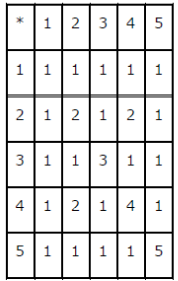# Consider a binary operation * on the set {1, 2, 3, 4, 5} given by the following multiplication table

Consider a binary operation * on the set {1, 2, 3, 4, 5} given by the following multiplication table (table 1.2).
(i) Compute (2 * 3) * 4 and 2 * (3 * 4)
(ii) Is * commutative?
(iii) Compute (2 * 3) * (4 * 5)
(Hint: Use the following table)